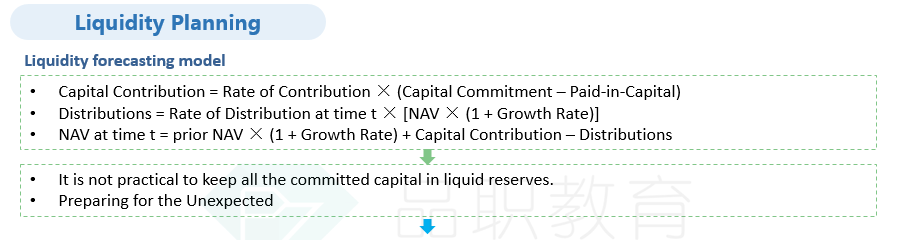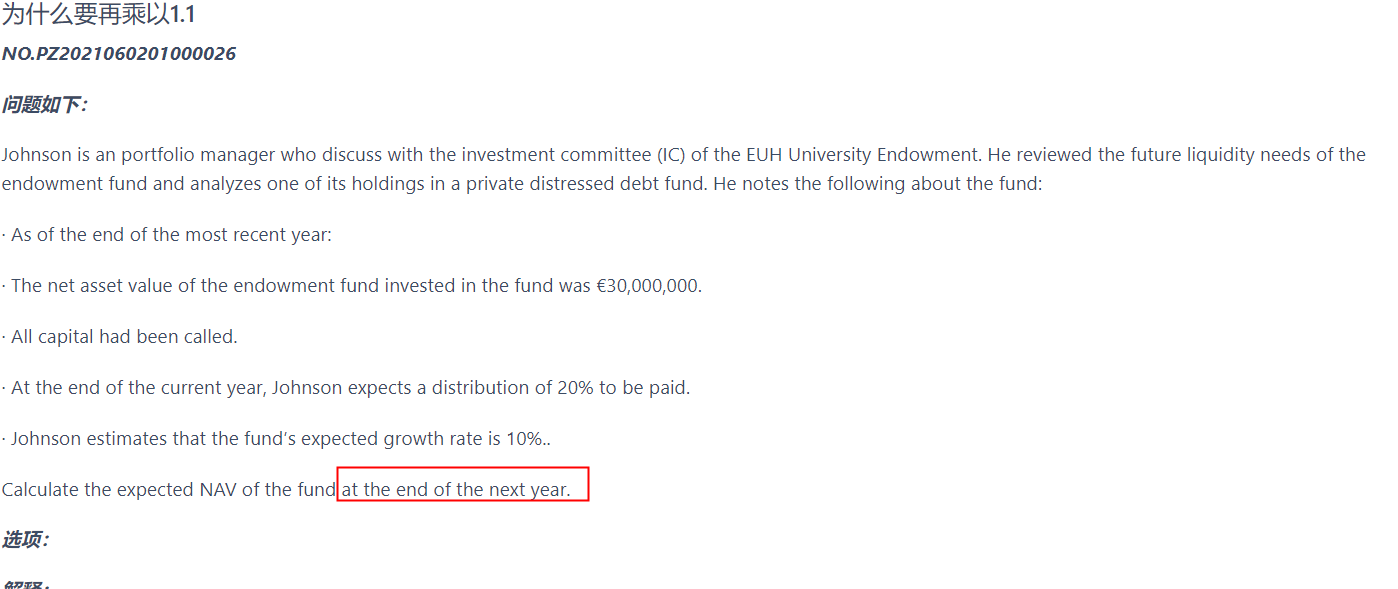# 为什么要再乘以1.1

NO.PZ2021060201000026

Johnson is an portfolio manager who discuss with the investment committee (IC) of the EUH University Endowment. He reviewed the future liquidity needs of the endowment fund and analyzes one of its holdings in a private distressed debt fund. He notes the following about the fund:

· As of the end of the most recent year:

· The net asset value of the endowment fund invested in the fund was €30,000,000.

· All capital had been called.

· At the end of the current year, Johnson expects a distribution of 20% to be paid.

· Johnson estimates that the fund’s expected growth rate is 10%..

Calculate the expected NAV of the fund at the end of the next year.

The expected NAV of the fund at the end of the next year is €29,040,000, calculated as follows:

First, the expected distribution at the end of the current year is calculated as

Expected distribution = [Prior-year NAV × (1 + Growth rate)] × (Distribution rate).

Expected distribution = [(€30,000,000 × 1.1) × 20%] = €6,600,000.

Therefore, the expected NAV of the fund at the end of the next year is

Expected NAV = [Prior-year NAV × (1 + Growth rate) + Capital contributions – Distributions)] × (1 + Growth rate).

Expected NAV = [(€30,000,000 × 1.1) + 0 − €6,600,000] × 1.1 = €29,040,000.###### 1 个答案----------------------------------------------